# TRIGONOMETRIC FUNCTIONS OF ACUTE ANGLES By M Jaya

• Slides: 13
Download presentation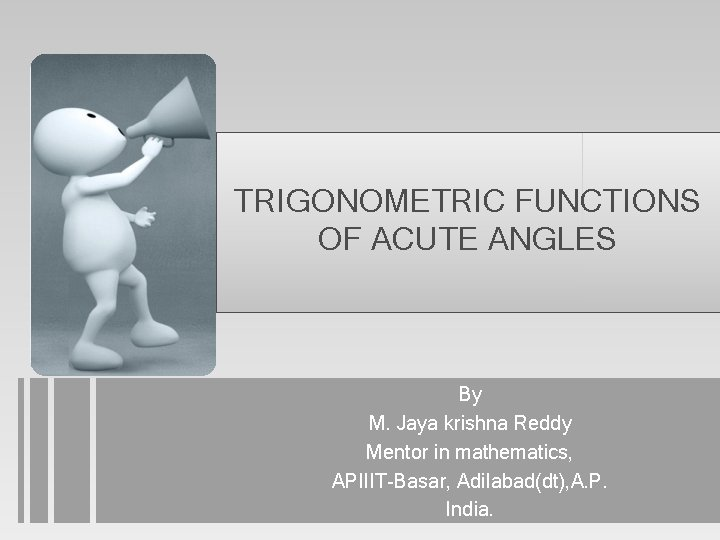TRIGONOMETRIC FUNCTIONS OF ACUTE ANGLES By M. Jaya krishna Reddy Mentor in mathematics, APIIIT-Basar, Adilabad(dt), A. P. India.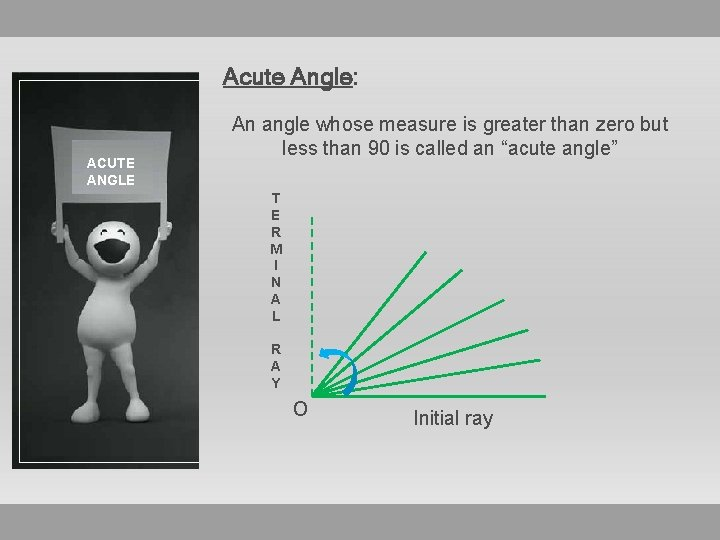Acute Angle: ACUTE ANGLE An angle whose measure is greater than zero but less than 90 is called an “acute angle” T E R M I N A L R A Y o Initial ray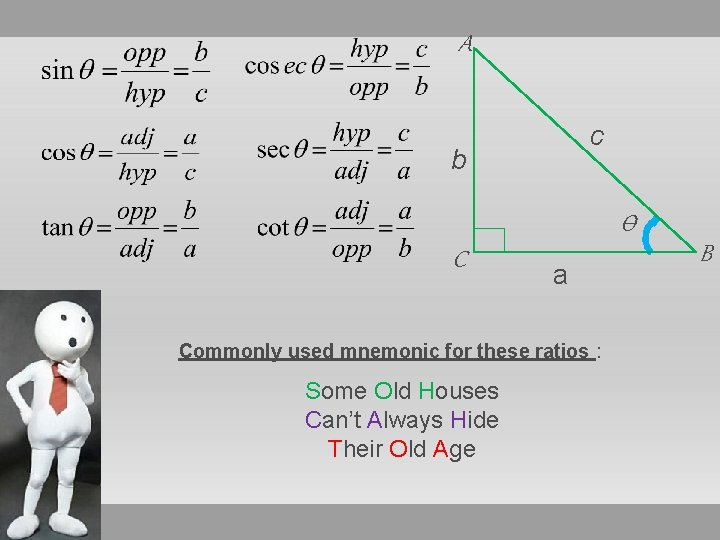A c b Ѳ C a Commonly used mnemonic for these ratios : Some Old Houses Can’t Always Hide Their Old Age BHistory: • Trigonometric functions(also called circular functions) are functions of an angle. • They are used to relate the angles of a triangle to the lengths of the sides of a triangle. • Sumerian astronomers introduced angle measure, using a division of circles into 360 degrees. • The sine function was first defined in the “surya siddhanta” and its properties were further documented by the fifth century Indian mathematician and astronomer “Aryabhatta”. • By 10 th century the six trigonometric functions were used.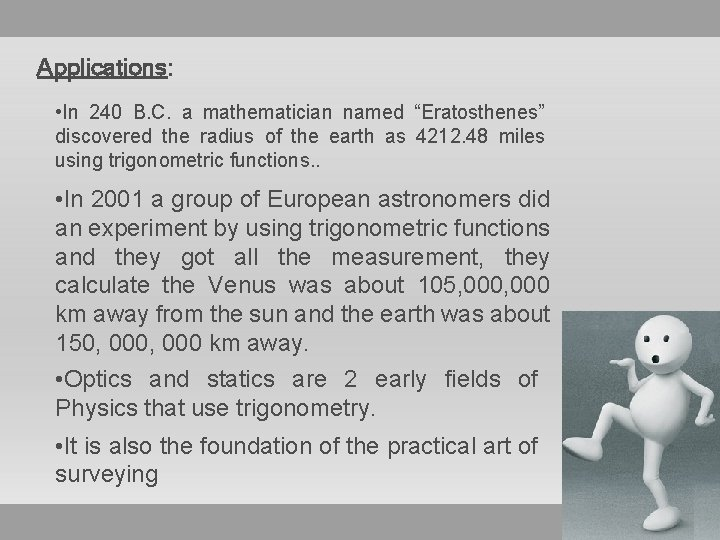Applications: • In 240 B. C. a mathematician named “Eratosthenes” discovered the radius of the earth as 4212. 48 miles using trigonometric functions. . • In 2001 a group of European astronomers did an experiment by using trigonometric functions and they got all the measurement, they calculate the Venus was about 105, 000 km away from the sun and the earth was about 150, 000 km away. • Optics and statics are 2 early fields of Physics that use trigonometry. • It is also the foundation of the practical art of surveying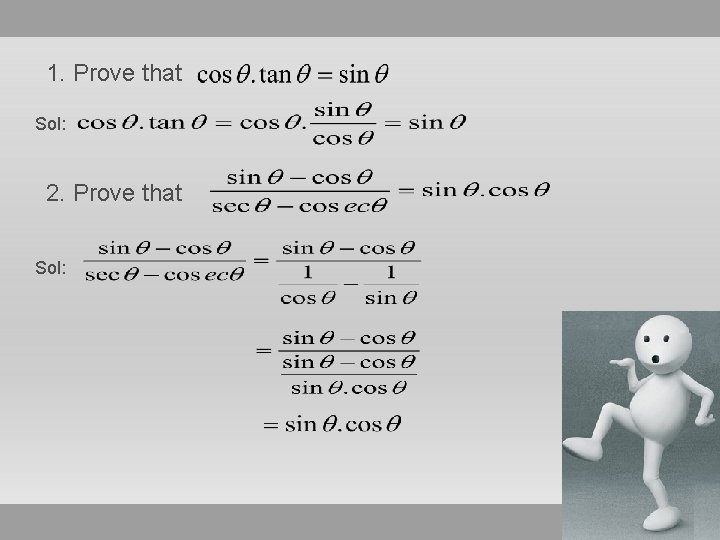1. Prove that Sol: 2. Prove that Sol:Fundamental Relations: A c b Ѳ C Squaring and adding both the equations a From the above diagram, By Pythagorean Rule, BA c b Squaring and subtracting the equations, we get Ѳ C a From the above diagram, By Pythagorean Rule, Similarly, B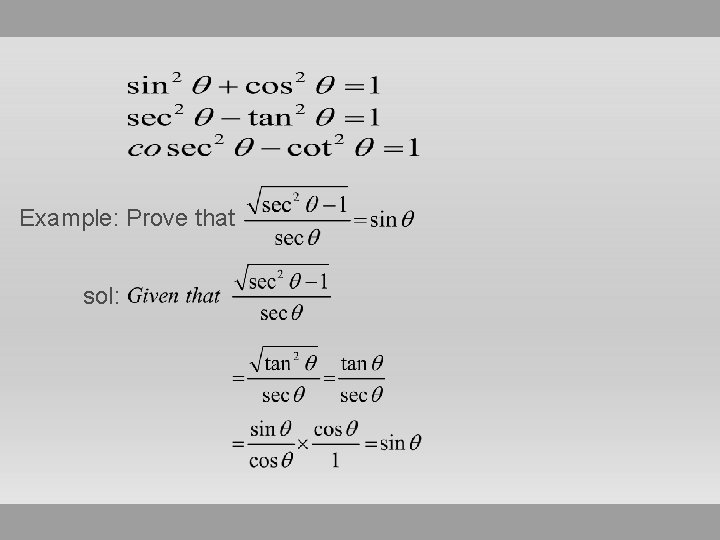Example: Prove that sol:Ex: Prove that sec 2Ѳ - cosec 2Ѳ = tan 2Ѳ - cot 2Ѳ Sol: We know that sec 2Ѳ - tan 2Ѳ = 1 = cosec 2Ѳ - cot 2Ѳ sec 2Ѳ - tan 2Ѳ = cosec 2Ѳ - cot 2Ѳ sec 2Ѳ - cosec 2Ѳ = tan 2Ѳ - cot 2Ѳ Example: Prove that Sol: Given that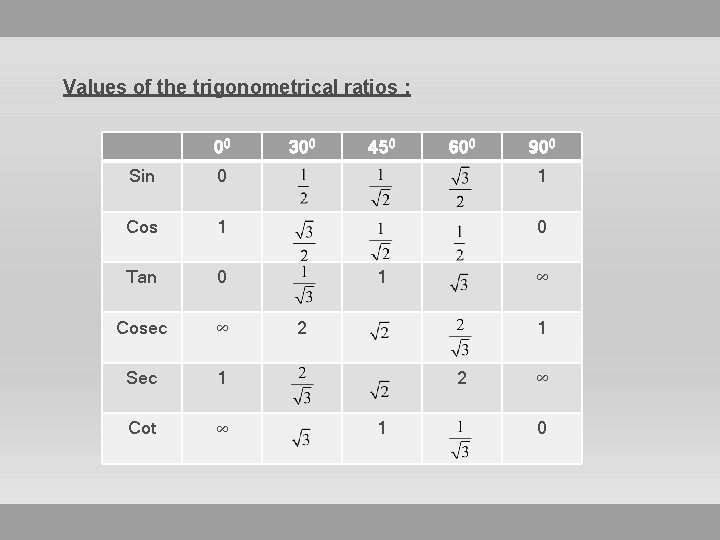Values of the trigonometrical ratios : 00 300 450 600 900 Sin 0 1 Cos 1 0 Tan 0 Cosec ∞ Sec 1 Cot ∞ 1 ∞ 2 1 ∞ 0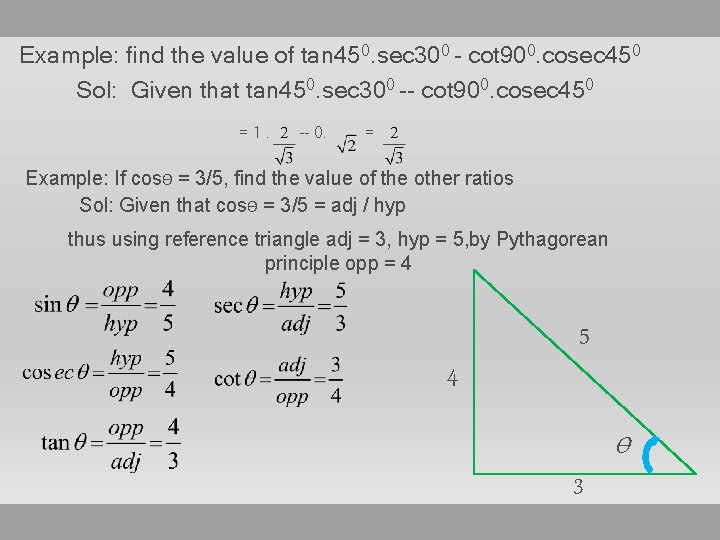Example: find the value of tan 450. sec 300 - cot 900. cosec 450 Sol: Given that tan 450. sec 300 -- cot 900. cosec 450 =1. -- 0. = Example: If cosѲ = 3/5, find the value of the other ratios Sol: Given that cosѲ = 3/5 = adj / hyp thus using reference triangle adj = 3, hyp = 5, by Pythagorean principle opp = 4 5 4 Ѳ 3THANK YOU